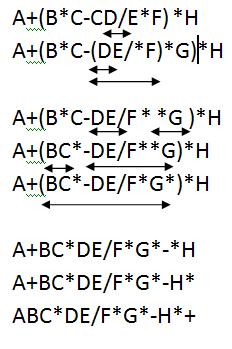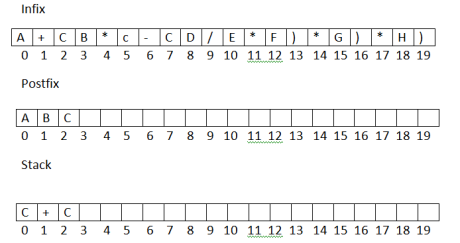Data Structures Tutorial
Ratings:
(4.3)
Views:2604## DataStructures Tutorial Overview

Welcome to the DataStructures Tutorial! The objective of these tutorials is to help you gain a solid understanding of data structures and their applications. By the end of these tutorials, you will be able to use data structures effectively to write efficient, scalable, and error-free code.

We will cover a wide range of topics, including stacks, sorting algorithms, queues, trees, graphs, and more. Each of these data structures has its unique properties, applications, and algorithms, and understanding them will help you write better programs.

In addition to the core concepts, we will also cover common interview questions related to data structures. Understanding these questions and how to solve them will help you ace technical interviews and build your confidence.

We will also address common issues that programmers face when working with data structures, such as memory management, performance optimization, and code maintainability.

Whether you are a beginner or an experienced programmer, this tutorial series will provide you with the knowledge and skills you need to master data structures and write better code. Let's get started!

## Data Structures

In computer science, a data structure is a particular way and organizing data in a computer so that it can be used efficiently.

A data structure is an arrangement of data in a computer memory or even disk storage.

An example of several common data structures are arrays, linked lists, queues, stacks, binary trees, and hash tables.

Different kinds of data structures are suited to different kinds of applications and some are highly specialized to specific basis.

For example, 3-trees are particular well suited for the implementation of databases, while compiler implementation usually use hash tables to look up identifiers.

Data structures are used in almost every program or software system.

Specific data structures are essential ingredients of many efficient algorithms and make possible the management of huge amounts of data, such as large databases and internet indexing services.

The following are the two types of the DataStructures:

1. Linear DataStructures.
2. Non-linear DataStructures.

### Linear Data Structures

Linear data structures store and organize data elements in a linear or sequential manner. These structures are often used for fast access and easy manipulation of data.

There are several types of linear data structures, including arrays, stacks, queues, and linked lists.

### Non-linear DataStructures

The non-linear DataStructures holds/stores the data elements in the form hierarchy by establishing some relationship between the data elements.

Trees and graphs are nonlinear data structures.

## Infix to Postfix conversions

In general, simple architecture expression can be represented in three ways: infix, prefix and postfix.

• Consider the expression A+B. Here A and B are operands and + is binary
• The three notations can be shown as :

A+B             infix +AB             prefix(or polish) AB+             postfix(pr suffix or reverse polish)

• In infix notation, the operator symbol is between the two operands.
• In prefix, the operator symbol is placed before is its two operands.
• In postfix, the operator symbol is placed before is its two operands.
• In postfix, the operator symbol is placed after its two operands(i.e the operator follows both operands)

The infix operands – precedence is as follows

1. parenthesis
2. multiplication * , Division

Algorithm:  In to post (Q, P) Suppose   ‘Q’  is an arithmetic expression written in infix notation. This algorithm finds the equivalent postfix expression p.

1. Push a left parenthesis onto the stack, and add a right parenthesis to the end of Q.
2. Scan Q from left to right and repeat steps 3 to 6 for each element of Q until the stack is empty.
3. If an operand is encountered, add it to the postfix expression P.
4. If a left parenthesis is encountered, push it onto the stack.
5. If an operator X is encountered, then:
• Repeatedly pop from the stack and add to P each operator on the top of the stack, which has the same precedence as or higher precedence than X.
• Push X onto the stack.
6. If a right parenthesis is encountered, then:
• Repeatedly pop from the stack and add to P each operator on the top of the stack until a left parenthesis is encountered.
• Pop the left parenthesis from the stack.
7. Exit

The algorithm works by using a stack to keep track of the operators encountered in the infix expression. When an operator is encountered, it is compared with the operator at the top of the stack. If the operator has higher or equal precedence than the one on the top of the stack, it is pushed onto the stack. Otherwise, the operator on top of the stack is popped and added to the postfix expression until an operator with lower precedence is encountered. The left and right parentheses are used to ensure that the order of evaluation is preserved.

Overall, the infix-to-postfix conversion algorithm is a useful technique for parsing arithmetic expressions and evaluating them in a more efficient manner.

Example : Q: A+(B*C-(D/E*F)*G)*H)

 Symbol Scanned STACK postfix(p) A C A + C+ A C C+C A B C+C AB * C+C* AB C C+C* ABC - C+C- ABC* ( C+C-C ABC*D 0 C+C-C ABC*D / C+C-C/ ABC*D E C+C-C/ ABC*DE * C+C-C ABC*DE/ F C+C-C* ABC*DE/F ) C+C- ABC*DE/F* * C+C- ABC*DE/F* G C+C-* ABC*DE/F*G ) C+C. ABC*DE/F*G*- * C+* ABC*DE/F*G*- H C+* ABC*DE/F*G*-H ) EMPTY ABC*DE/F*G*-H*+### Program to convert infix to postfix expressions

``````#include<stdio.h>
#include<conio.h>
#include<ctype.h>
#include<stdlib.h>

char infix;
char postfix;
char stack;
int TOP=-1;

void GetExpr() {
int len;
printf("Enter an infix expression:\n");
gets(infix);
len = strlen(infix);
infix[len] = ')';
TOP = TOP + 1;
stack[TOP] = 'c';
}

void convert() {
int i, j;
for(i = 0, j = 0; TOP != -1; i++) {
if(isalpha(infix[i])) {
postfix[j] = infix[i];
j = j + 1;
}
else {
switch(infix[i]) {
case '(':
TOP = TOP + 1;
stack[TOP] = '(';
break;
case '+':
case '-':
while(stack[TOP] == '+' || stack[TOP] == '-' || stack[TOP] == '*' || stack[TOP] == '/') {
postfix[j] = stack[TOP];
j = j + 1;
TOP = TOP - 1;
}
TOP = TOP + 1;
stack[TOP] = infix[i];
break;
case '*':
case '/':
while(stack[TOP] == '*' || stack[TOP] == '/') {
postfix[j] = stack[TOP];
j = j + 1;
TOP = TOP - 1;
}
TOP = TOP + 1;
stack[TOP] = infix[i];
break;
case ')':
while(stack[TOP] != '(' && (stack[TOP] == '+' || stack[TOP] == '-' || stack[TOP] == '*' || stack[TOP] == '/')) {
postfix[j] = stack[TOP];
j = j + 1;
TOP = TOP - 1;
}
TOP = TOP - 1;
break;
} /* switch end */
} /* else end */
} /* for end */
postfix[j] = '\0';
printf("Expression in postfix is %s\n", postfix);
}

int main() {
clrscr();
GetExpr();
convert();
getch();
return 0;
}
``````

Output:  Enter an expression A+(B*C  - CDIE*F)